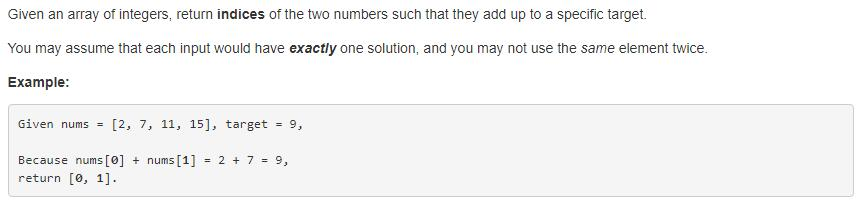## 1. 题目描述 （简单难度）## 2. 解法一

public int[] twoSum(int[] nums, int target) {
int []ans=new int;
for(int i=0;i<nums.length;i++){
for(int j=(i+1);j<nums.length;j++){
if(nums[i]+nums[j]==target){
ans=i;
ans=j;
return ans;
}
}
}
return ans;
}


## 3. 解法二

for(int j=(i+1);j<nums.length;j++){
if(nums[i]+nums[j]==target){


for(int j=(i+1);j<nums.length;j++){
sub=target-nums[i]
if(nums[j]==sub){


hash table ！！！

public int[] twoSum(int[] nums, int target) {
Map<Integer,Integer> map=new HashMap<>();
for(int i=0;i<nums.length;i++){
map.put(nums[i],i);
}
for(int i=0;i<nums.length;i++){
int sub=target-nums[i];
if(map.containsKey(sub)&&map.get(sub)!=i){
return new int[]{i,map.get(sub)};
}
}
throw new IllegalArgumentException("No two sum solution");
}


## 4. 解法三

public int[] twoSum(int[] nums, int target) {
Map<Integer,Integer> map=new HashMap<>();
for(int i=0;i<nums.length;i++){
int sub=target-nums[i];
if(map.containsKey(sub)){
return new int[]{i,map.get(sub)};
}
map.put(nums[i], i);
}
throw new IllegalArgumentException("No two sum solution");
}